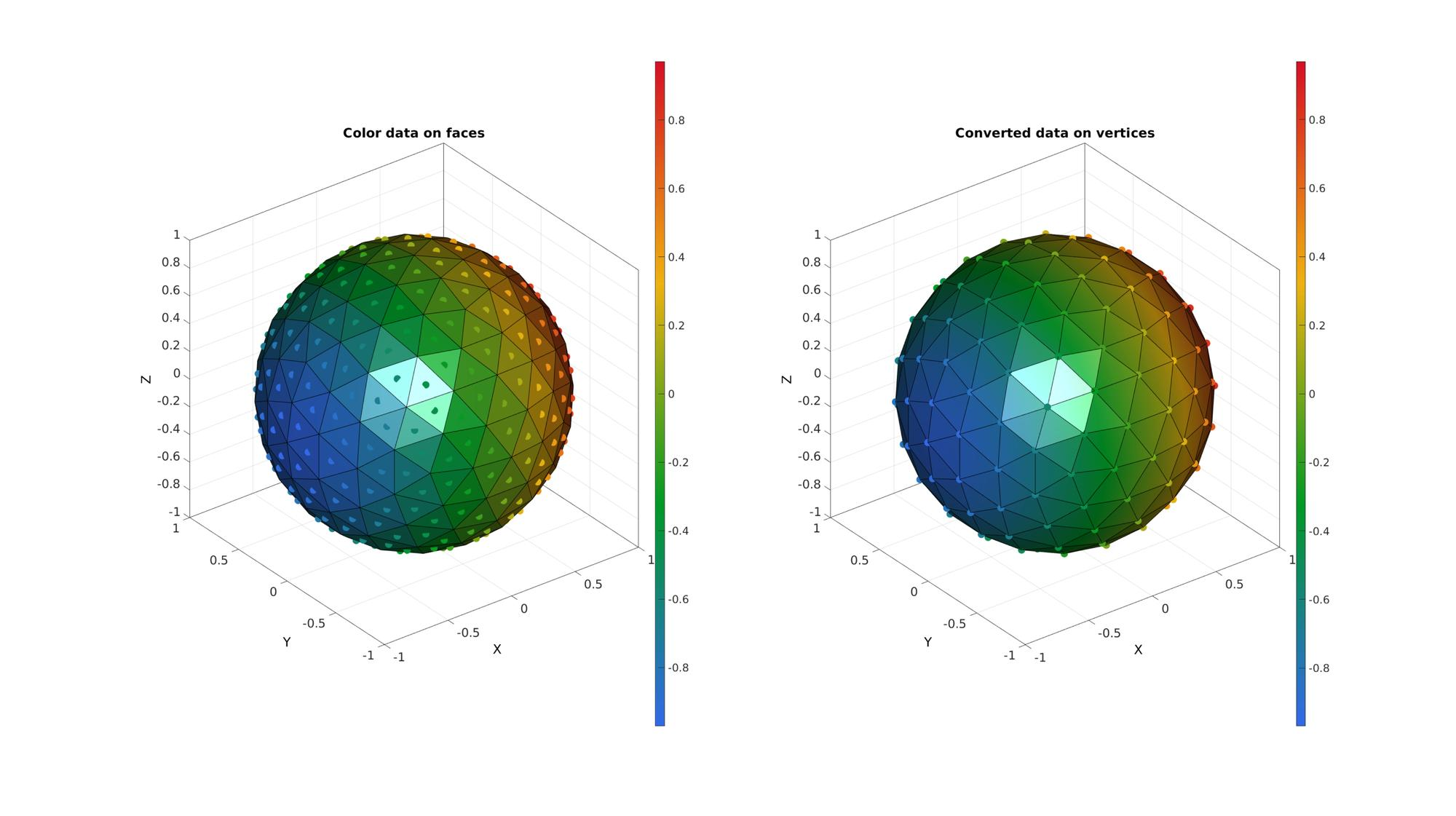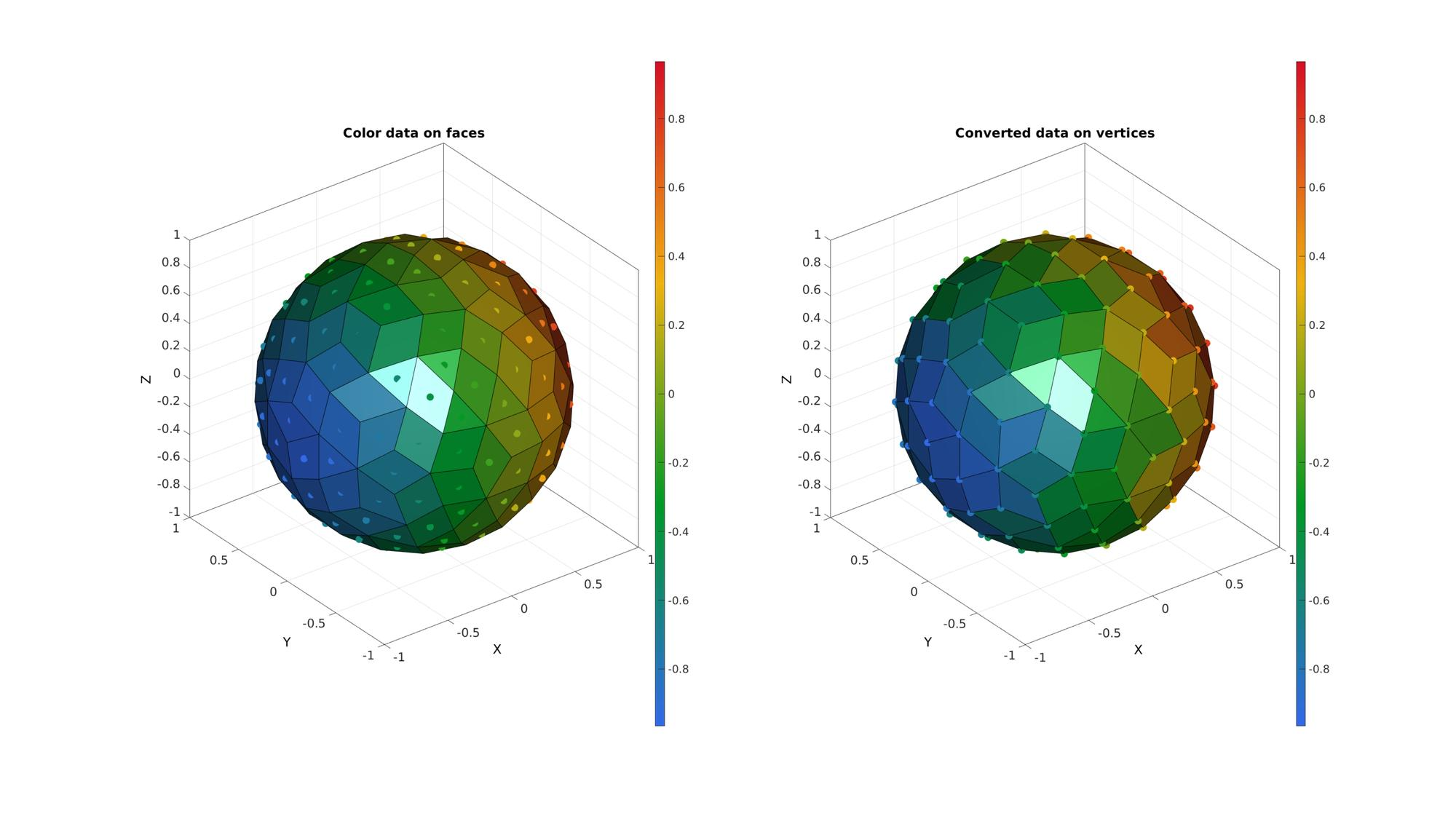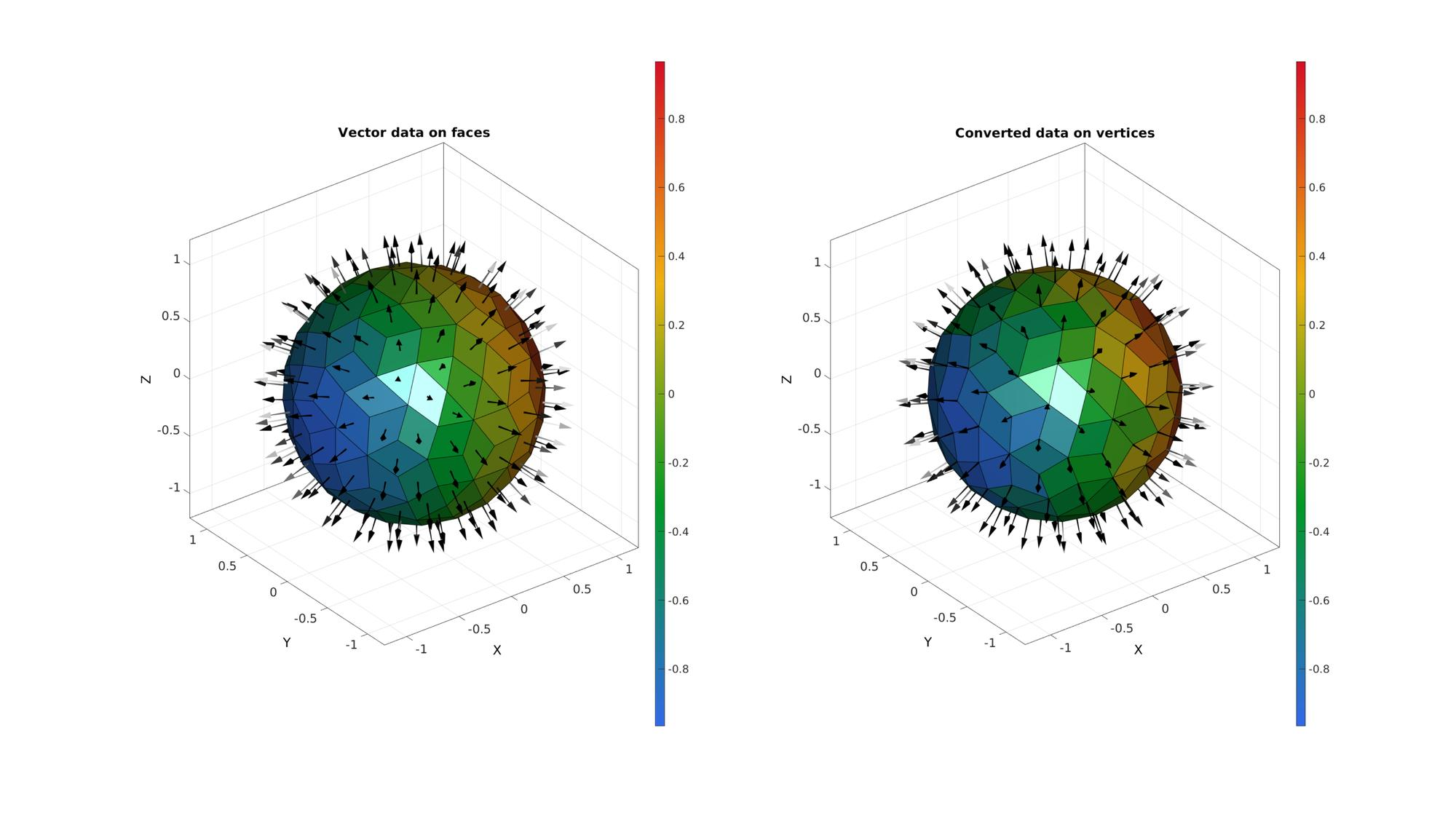# faceToVertexMeasure

Below is a demonstration of the features of the patchPathAngles function

## Contents

```clear; close all; clc;
```

## Syntax

[indAngles]=faceToVertexMeasure(F,V,ind,isClosedLoop);

## Description

The faceToVertexMeasure function converts data for faces to data on vertices through averaging.

## Examples

Plot settings

```markerSize=150;
```

## Example 1: Convert face data to vertex data

```[F,V]=geoSphere(2,1);
VF=patchCentre(F,V);
CF=VF(:,1);
[CV]=faceToVertexMeasure(F,V,CF);
```
```cFigure;
subplot(1,2,1); hold on;
title('Color data on faces')
gpatch(F,V,CF);
scatterV(VF,markerSize,CF,'filled');
colormap gjet; colorbar;
axisGeom;

subplot(1,2,2); hold on;
title('Converted data on vertices')
hp=gpatch(F,V,CV);
hp.FaceColor='Interp';
scatterV(V,markerSize,CV,'filled');
colormap gjet; colorbar;
axisGeom;

drawnow;
```## Example 2: Convert face data on a mixed mesh to vertex data

Create an example of a mixed mesh consisting of triangles and quadrilateral faces.

```% Create a triangular mesh
[F,V]=geoSphere(2,1);

% Converting to a quadrilateral mesh
optionStruct.maxAngleDeviation=45*(pi/180);
optionStruct.selectionMethod='best';
optionStruct.triangleConvert=0;
optionStruct.fourConnectConvert=0;

VF=patchCentre(F,V);
CF=VF;
for q=1:1:numel(VF)
CF{q}=VF{q}(:,1);
end
[CV]=faceToVertexMeasure(F,V,CF);
```
```cFigure;
subplot(1,2,1); hold on;
title('Color data on faces')
gpatch(F,V,CF);
for q=1:1:numel(VF)
scatterV(VF{q},markerSize,CF{q},'filled');
end
colormap gjet; colorbar;
axisGeom;

subplot(1,2,2); hold on;
title('Converted data on vertices')
hp=gpatch(F,V,CV);
% hp.FaceColor='Interp';
scatterV(V,markerSize,CV,'filled');
colormap gjet; colorbar;
axisGeom;
drawnow;
```## Example 3: Convert multi-dimensional face data (e.g. on a mixed mesh) to vertex data

```N=patchNormal(F,V); %get face normals
NV=faceToVertexMeasure(F,V,N); %Convert to vertex normals
```
```VF=patchCentre(F,V); %Get face centres for plotting

cFigure;
subplot(1,2,1); hold on;
title('Vector data on faces')
gpatch(F,V,CF);
for q=1:1:numel(F)
quiverVec(VF{q},N{q},0.25,'k');
end
colormap gjet; colorbar;
axisGeom;

subplot(1,2,2); hold on;
title('Converted data on vertices')
gpatch(F,V,CV);
quiverVec(V,NV,0.25,'k');
colormap gjet; colorbar;
axisGeom;
drawnow;
```GIBBON www.gibboncode.org

Kevin Mattheus Moerman, [email protected]

GIBBON footer text

GIBBON: The Geometry and Image-based Bioengineering add-On. A toolbox for image segmentation, image-based modeling, meshing, and finite element analysis.

Copyright (C) 2019 Kevin Mattheus Moerman

This program is free software: you can redistribute it and/or modify it under the terms of the GNU General Public License as published by the Free Software Foundation, either version 3 of the License, or (at your option) any later version.

This program is distributed in the hope that it will be useful, but WITHOUT ANY WARRANTY; without even the implied warranty of MERCHANTABILITY or FITNESS FOR A PARTICULAR PURPOSE. See the GNU General Public License for more details.

You should have received a copy of the GNU General Public License along with this program. If not, see http://www.gnu.org/licenses/.